Browse by Topic

# Cultivating New Solutions for the Orchard-Planting Problem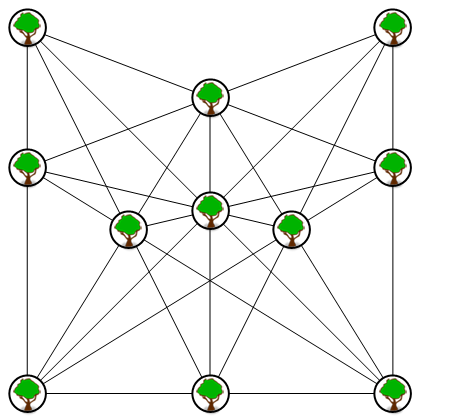Some trees are planted in an orchard. What is the maximum possible number of distinct lines of three trees? In his 1821 book Rational Amusement for Winter Evenings, J. Jackson put it this way:

Fain would I plant a grove in rows
But how must I its form compose
With three trees in each row;
To have as many rows as trees;
Now tell me, artists, if you please:
‘Tis all I want to know.

Those familiar with tic-tac-toe, three-in-a-row might wonder how difficult this problem could be, but it’s actually been looked at by some of the most prominent mathematicians of the past and present. This essay presents many new solutions that haven’t been seen before, shows a general method for finding more solutions and points out where current best solutions are improvable.

Various classic problems in recreational mathematics are of this type:

1. Plant 7 trees to make 6 lines of 3 trees.
2. Plant 8 trees to make 7 lines of 3 trees.
3. Plant 9 trees to make 10 lines of 3 trees.
4. Plant 10 trees to make 12 lines of 3 trees.
5. Plant 11 trees to make 16 lines of 3 trees.

Here is a graphic for the last problem, 11 trees with 16 lines of 3 trees. Subsets[points,{3}] collects all sets of 3 points. Abs[Det[Append[#,1]&/@#]] calculates the triangle area of each set. The sets with area 0 are the lines.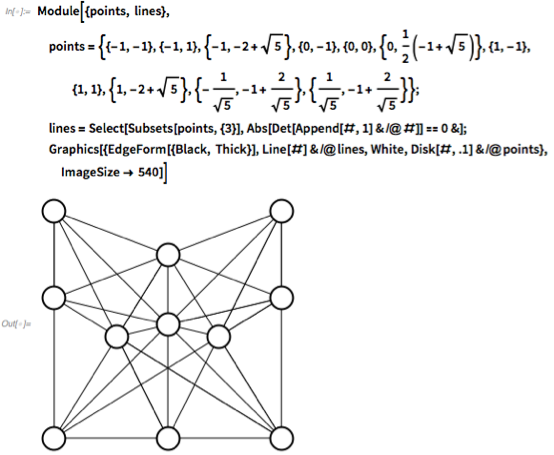✕ ```Module[{points, lines}, points = {{-1, -1}, {-1, 1}, {-1, -2 + Sqrt}, {0, -1}, {0, 0}, {0, 1/2 (-1 + Sqrt)}, {1, -1}, {1, 1}, {1, -2 + Sqrt}, {-(1/Sqrt), -1 + 2/Sqrt}, {1/Sqrt[ 5], -1 + 2/Sqrt}}; lines = Select[Subsets[points, {3}], Abs[Det[Append[#, 1] & /@ #]] == 0 &]; Graphics[{EdgeForm[{Black, Thick}], Line[#] & /@ lines, White, Disk[#, .1] & /@ points}, ImageSize -> 540]]```

This solution for 12 points matches the known limit of 19 lines, but uses simple integer coordinates and seems to be new. Lines are found with GatherBy and RowReduce, which quickly find a canonical line form for any 2 points in either 2D or 3D space.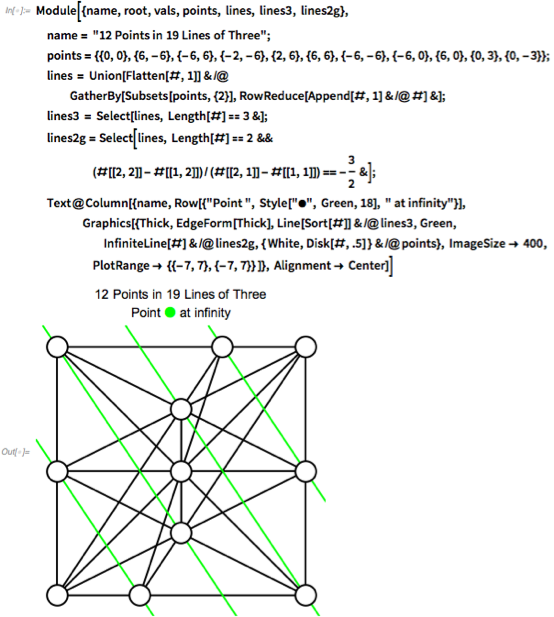✕ ```Module[{name, root, vals, points, lines, lines3, lines2g}, name = "12 Points in 19 Lines of Three"; points = {{0, 0}, {6, -6}, {-6, 6}, {-2, -6}, {2, 6}, {6, 6}, {-6, -6}, {-6, 0}, {6, 0}, {0, 3}, {0, -3}}; lines = Union[Flatten[#, 1]] & /@ GatherBy[Subsets[points, {2}], RowReduce[Append[#, 1] & /@ #] &]; lines3 = Select[lines, Length[#] == 3 &]; lines2g = Select[lines, Length[#] == 2 && (#[[2, 2]] - #[[1, 2]])/(#[[2, 1]] - #[[1, 1]]) == -(3/2) &]; Text@Column[{name, Row[{"Point ", Style["\[FilledCircle]", Green, 18], " at infinity"}], Graphics[{Thick, EdgeForm[Thick], Line[Sort[#]] & /@ lines3, Green, InfiniteLine[#] & /@ lines2g, { White, Disk[#, .5] } & /@ points}, ImageSize -> 400, PlotRange -> {{-7, 7}, {-7, 7}} ]}, Alignment -> Center]]```

This blog goes far beyond those old problems. Here’s how 27 points can make 109 lines of 3 points. If you’d like to see the best-known solutions for 7 to 27 points, skip to the gallery of solutions at the end. For the math, code and methodology behind these solutions, keep reading.✕ ```With[{n = 27}, Quiet@zerosumGraphic[ If[orchardsolutions[[n, 2]] > orchardsolutions[[n, 3]], orchardsolutions[[n, 6]], Quiet@zerotripsymm[orchardsolutions[[n, 4]], Floor[(n - 1)/2]]], n, {260, 210} 2]]```

What is the behavior as the number of trees increases? MathWorld’s orchard-planting problem, Wikipedia’s orchard-planting problem and the On-Line Encyclopedia of Integer Sequences sequence A003035 list some of what is known. Let m be the number of lines containing exactly three points for a set of p points. In 1974, Burr, Grünbaum and Sloane (BGS) gave solutions for particular cases and proved the bounds: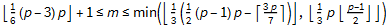Here’s a table.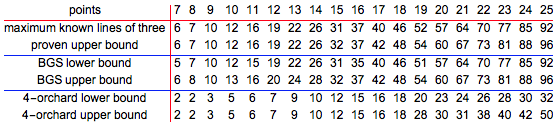✕ ```droppoints = 3; Style[Text@Grid[Transpose[ Drop[Prepend[ Transpose[{Range[7, 28], Drop[#[] & /@ orchardsolutions, 6], {6, 7, 10, 12, 16, 19, 22, 26, 32, 37, 42, 48, 54, 60, 67, 73, 81, 88, 96, 104, 113, 121}, (Floor[# (# - 3)/6] + 1) & /@ Range[7, 28], Min[{Floor[#/3 Floor[(# - 1)/2]], Floor[(Binomial[#, 2] - Ceiling[3 #/7])/3]}] & /@ Range[7, 28], {2, 2, 3, 5, 6, 7, 9, 10, 12, 15, 16, 18, 20, 23, 24, 26, 28, 30, 32, "?", "?", "?"}, {2, 2, 3, 5, 6, 7, 9, 10, 12, 15, 16, 18, 28, 30, 31, 38, 40, 42, 50, "?", "?", "?"} }], {"points", "maximum known lines of three", "proven upper bound", "BGS lower bound", "BGS upper bound", "4-orchard lower bound", "4-orchard upper bound"}], -droppoints]], Dividers -> {{2 -> Red}, {2 -> Red, 4 -> Blue, 6 -> Blue}}], 12]```

Terence Tao and Ben Green recently proved that the maximum number of lines is the BGS lower bound most of the time (“On Sets Defining Few Ordinary Lines”), but they did not describe how to get the sporadic exceptions. Existing literature does not currently show the more complicated solutions. For this blog, I share a method for getting elegant-looking solutions for the three-orchard problem, as well as describing and demonstrating the power of a method for finding the sporadic solutions. Most of the embeddings shown in this blog are new, but they all match existing known records.

For a given number of points p, let q = ⌊ (p–1)/2⌋; select the 3-subsets of {–q,–q+1,…,q} that have a sum of 0 (mod p). That gives ⌊ (p–3) p/6⌋+1 3-subsets. Here are the triples from p=8 to p=14. This number of triples is the same as the lower bound for the orchard problem, which Tao and Green proved was the best solution most of the time.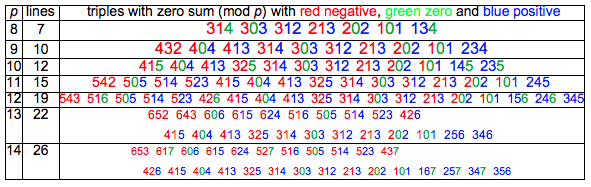✕ ```Text@Grid[Prepend[Table[With[{triples = Select[ Subsets[Range[-Floor[(p - 1)/2], Ceiling[(p - 1)/2]], {3}], Mod[Total[#], p] == 0 &]}, {p, Length[triples], Row[Row[ Text@Style[ ToString[Abs[#]], {Red, Darker[Green], Blue}[[ If[# == p/2, 2, Sign[#] + 2]]], 25 - p] & /@ #] & /@ triples, Spacer]}], {p, 8, 14}], {" \!\(\* StyleBox[\"p\",\nFontSlant->\"Italic\"]\)\!\(\* StyleBox[\" \",\nFontSlant->\"Italic\"]\)", " lines ", Row[ {" triples with zero sum (mod \!\(\* StyleBox[\"p\",\nFontSlant->\"Italic\"]\)) with \!\(\* StyleBox[\"red\",\nFontColor->RGBColor[1, 0, 0]]\)\!\(\* StyleBox[\" \",\nFontColor->RGBColor[1, 0, 0]]\)\!\(\* StyleBox[\"negative\",\nFontColor->RGBColor[1, 0, 0]]\), \!\(\* StyleBox[\"green\",\nFontColor->RGBColor[0, 1, 0]]\)\!\(\* StyleBox[\" \",\nFontColor->RGBColor[0, 1, 0]]\)\!\(\* StyleBox[\"zero\",\nFontColor->RGBColor[0, 1, 0]]\) and \!\(\* StyleBox[\"blue\",\nFontColor->RGBColor[0, 0, 1]]\)\!\(\* StyleBox[\" \",\nFontColor->RGBColor[0, 0, 1]]\)\!\(\* StyleBox[\"positive\",\nFontColor->RGBColor[0, 0, 1]]\)"}]}], Spacings -> {0, 0}, Frame -> All]```

Here’s a clearer graphic for how this works. Pick three different numbers from –8 to 8 that have a sum of zero. You will find that those numbers are on a straight line. The method used to place these numbers will come later.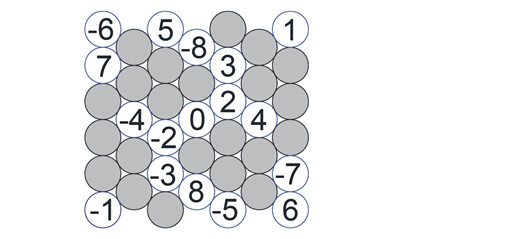That’s not the maximum possible number of lines. By moving these points some, the triples that have a modulus-17 sum of zero can also be lines. One example is 4 + 6 + 7 = 17.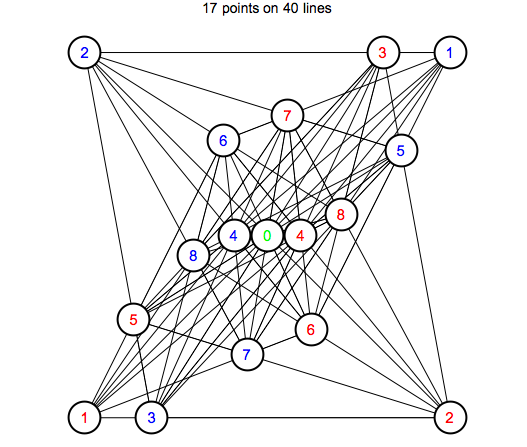✕ ```With[{n = 17}, Quiet@zerosumGraphic[ If[orchardsolutions[[n, 2]] > orchardsolutions[[n, 3]], orchardsolutions[[n, 6]], Quiet@zerotripsymm[orchardsolutions[[n, 4]], Floor[(n - 1)/2]]], n, {260, 210} 2]]```

Does this method always give the best solution? No—there are at least four sporadic exceptions. Whether any other sporadic solutions exist is not known.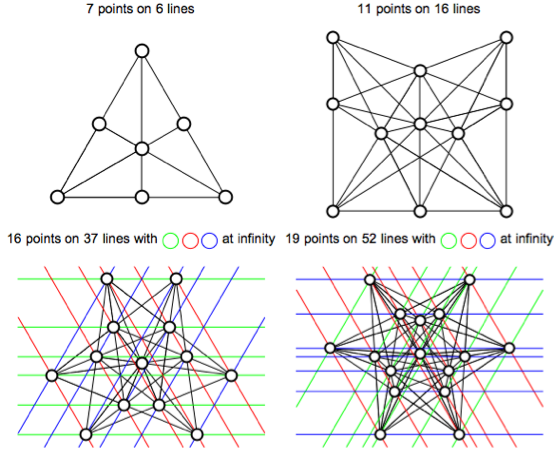✕ ```Grid[Partition[ zerosumGraphic[orchardsolutions[[#, 6]], #, {260, 210}] & /@ {7, 11, 16, 19}, 2]]```

## More Than Three in a Row

There are also problems with more than three in a row.

1. Plant 16 trees to make 15 lines of 4 trees.
2. Plant 18 trees to make 18 lines of 4 trees.
3. Plant 25 trees in 18 lines of 5 points.
4. Plant 112 trees in 3D to make 27 lines of 7 trees.

Fifteen lines of four points using 15 points is simple enough. RowReduce is used to collect lines, with RootReduce added to make sure everything is in a canonical form.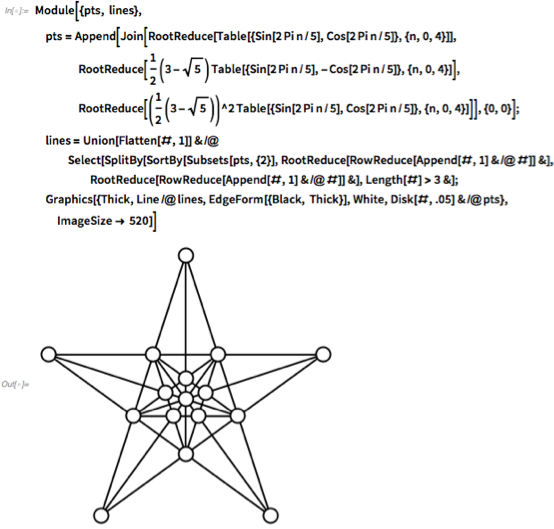✕ ```Module[{pts, lines}, pts = Append[ Join[RootReduce[Table[{Sin[2 Pi n/5], Cos[2 Pi n/5]}, {n, 0, 4}]], RootReduce[ 1/2 (3 - Sqrt) Table[{Sin[2 Pi n/5], -Cos[2 Pi n/5]}, {n, 0, 4}]], RootReduce[(1/2 (3 - Sqrt))^2 Table[{Sin[2 Pi n/5], Cos[2 Pi n/5]}, {n, 0, 4}]]], {0, 0}]; lines = Union[Flatten[#, 1]] & /@ Select[SplitBy[ SortBy[Subsets[pts, {2}], RootReduce[RowReduce[Append[#, 1] & /@ #]] &], RootReduce[RowReduce[Append[#, 1] & /@ #]] &], Length[#] > 3 &]; Graphics[{Thick, Line /@ lines, EdgeForm[{Black, Thick}], White, Disk[#, .05] & /@ pts}, ImageSize -> 520]]```

Eighteen points in 18 lines of 4 points is harder, since it seems to require 3 points at infinity. When lines are parallel, projective geometers say that the lines intersect at infinity. With 4 points on each line and each line through 4 points, this is a 4-configuration.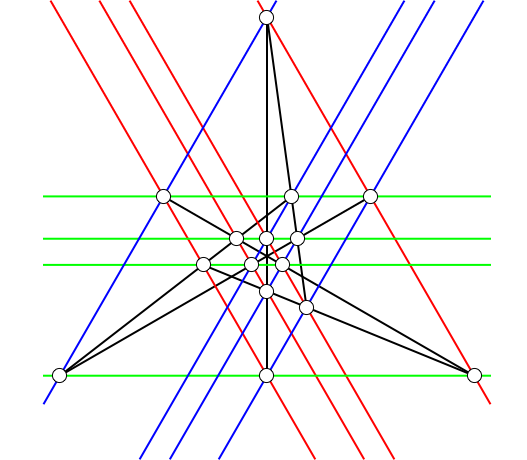✕ ```Module[{config18, linesconfig18, inf}, config18 = {{0, Root[9 - 141 #1^2 + #1^4 &, 1]}, {1/4 (-21 - 9 Sqrt), Root[9 - 564 #1^2 + 16 #1^4 &, 4]}, {1/4 (21 + 9 Sqrt), Root[9 - 564 #1^2 + 16 #1^4 &, 4]}, {0, -2 Sqrt}, {-3, Sqrt[ 3]}, {3, Sqrt}, {0, Sqrt}, {3/ 2, -(Sqrt/2)}, {-(3/2), -(Sqrt/2)}, {1/4 (3 + 3 Sqrt), Root[9 - 564 #1^2 + 16 #1^4 &, 4]}, {1/4 (9 + 3 Sqrt), Root[225 - 420 #1^2 + 16 #1^4 &, 1]}, {1/2 (-6 - 3 Sqrt), -( Sqrt/2)}, {0, Root[144 - 564 #1^2 + #1^4 &, 4]}, {1/2 (21 + 9 Sqrt), Root[9 - 141 #1^2 + #1^4 &, 1]}, {1/2 (-21 - 9 Sqrt), Root[9 - 141 #1^2 + #1^4 &, 1]}}; linesconfig18 = SplitBy[SortBy[Union[Flatten[First[#], 1]] & /@ (Transpose /@ Select[ SplitBy[ SortBy[{#, RootReduce[RowReduce[Append[#, 1] & /@ #]]} & /@ Subsets[config18, {2}], Last], Last], Length[#] > 1 &]), Length], Length]; inf = Select[ SplitBy[SortBy[linesconfig18[], RootReduce[slope[Take[#, 2]]] &], RootReduce[slope[Take[#, 2]]] &], Length[#] > 3 &]; Graphics[{Thick, Line /@ linesconfig18[], Red, InfiniteLine[Take[#, 2]] & /@ inf[], Green, InfiniteLine[Take[#, 2]] & /@ inf[], Blue, InfiniteLine[Take[#, 2]] & /@ inf[], EdgeForm[Black], White, Disk[#, .7] & /@ config18}, ImageSize -> {520, 460}]]```

If you do not like points at infinity, arrange 3 heptagons of 7 points to make a 4-configuration of 21 lines through 21 points. That isn’t the record, since it is possible to make at least 24 lines of 4 with 21 points.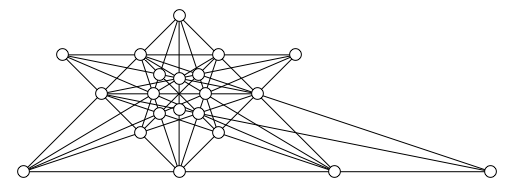✕ ```Module[{pts, lines}, 21 linepts = 4 {{0, -b}, {0, (b c)/( a - c)}, {2 a, -b}, {0, -((b c)/(2 a + c))}, {0, (b c)/( 3 a - c)}, {-a, -b}, {a, -b}, {-c, 0}, {-(c/3), 0}, {c/3, 0}, {c, 0}, {-((3 a c)/(3 a - 2 c)), (2 b c)/(3 a - 2 c)}, {( a c)/(3 a - 2 c), (2 b c)/(3 a - 2 c)}, {(3 a c)/(3 a - 2 c), ( 2 b c)/(3 a - 2 c)}, {(a c)/(5 a - 2 c), (2 b c)/( 5 a - 2 c)}, {(a c)/(-5 a + 2 c), (2 b c)/(5 a - 2 c)}, {( a c)/(-3 a + 2 c), (2 b c)/( 3 a - 2 c)}, {-((a c)/(a + 2 c)), -((2 b c)/(a + 2 c))}, {( a c)/(a + 2 c), -((2 b c)/(a + 2 c))}, {-((a c)/( 3 a + 2 c)), -((2 b c)/(3 a + 2 c))}, {(a c)/( 3 a + 2 c), -((2 b c)/(3 a + 2 c))}} /. {a -> 2, c -> 1, b -> 1}; lines = Union[Flatten[#, 1]] & /@ Select[SplitBy[ SortBy[Subsets[pts, {2}], RowReduce[Append[#, 1] & /@ #] &], RowReduce[Append[#, 1] & /@ #] &], Length[#] > 3 &]; Graphics[{Line /@ lines, EdgeForm[Black], White, Disk[#, .3] & /@ pts}, ImageSize -> 500]]```

The best-known solution for 25 points has 32 lines, but this solution seems weak due to the low contribution made by the last 3 points. Progressively remove points labeled 25, 24, 23 (near the bottom) to see the best-known solutions that produce 30, 28, 26 lines.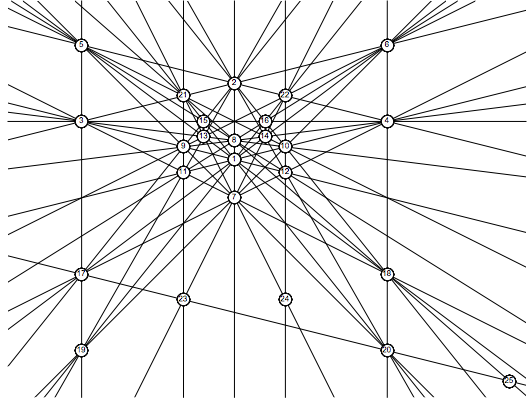✕ ```Module[{pts, lines}, pts = {{0, 1/4}, {0, 3/4}, {-1, 1/2}, {1, 1/2}, {-1, 1}, {1, 1}, {0, 0}, {0, 3/8}, {-(1/3), 1/3}, {1/3, 1/3}, {-(1/3), 1/6}, {1/3, 1/ 6}, {-(1/5), 2/5}, {1/5, 2/5}, {-(1/5), 1/2}, {1/5, 1/ 2}, {-1, -(1/2)}, {1, -(1/2)}, {-1, -1}, {1, -1}, {-(1/3), 2/ 3}, {1/3, 2/3}, {-(1/3), -(2/3)}, {1/3, -(2/3)}, {9/5, -(6/5)}}; lines = SplitBy[SortBy[ (Union[Flatten[#, 1]] & /@ SplitBy[SortBy[Subsets[pts, {2}], RowReduce[Append[#, 1] & /@ #] &], RowReduce[Append[#, 1] & /@ #] &]), Length], Length]; Graphics[{InfiniteLine[Take[#, 2]] & /@ lines[], White, EdgeForm[Black], Table[{Disk[pts[[n]], .04], Black, Style[Text[n, pts[[n]]], 8]}, {n, 1, Length[pts]}] & /@ pts, Black}, ImageSize -> {520}]]```

The 27 lines in space are, of course, the Clebsch surface. There are 12 points of intersection not shown, and some lines have 9 points of intersection.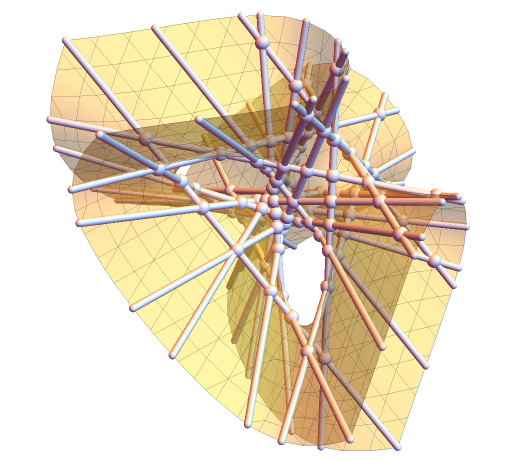✕ ```Module[{lines27, clebschpoints}, lines27 = Transpose /@ Flatten[Join[Table[RotateRight[#, n], {n, 0, 2}] & /@ {{{-(1/3), -(1/3)}, {1, -1}, {-1, 1}}, {{0, 0}, {1, -(2/3)}, {-(2/3), 1}}, {{1/3, 1/ 3}, {1, -(1/3)}, {-(1/3), 1}}, {{0, 0}, {4/ 9, -(2/9)}, {1, -1}}, {{0, 0}, {1, -1}, {4/9, -(2/9)}}}, Permutations[#] & /@ {{{30, -30}, {35 - 19 Sqrt, -25 + 17 Sqrt}, {5 + 3 Sqrt, 5 - 9 Sqrt}}/ 30, {{6, -6}, {-3 + 2 Sqrt, 6 - Sqrt}, {-7 + 4 Sqrt, 8 - 5 Sqrt}}/6}], 1]; clebschpoints = Union[RootReduce[Flatten[With[ {sol = Solve[e #[[1, 1]] + (1 - e) #[[1, 2]] == f #[[2, 1]] + (1 - f) #[[2, 2]]]}, If[Length[sol] > 0, (e #[[1, 1]] + (1 - e) #[[1, 2]]) /. sol, Sequence @@ {} ]] & /@ Subsets[lines27, {2}], 1]]]; Graphics3D[{{ Sphere[#, .04] & /@ Select[clebschpoints, Norm[#] < 1 &]}, Tube[#, .02] & /@ lines27, Opacity[.4], ContourPlot3D[ 81 (x^3 + y^3 + z^3) - 189 (x^2 y + x^2 z + x y^2 + x z^2 + y^2 z + y z^2) + 54 x y z + 126 (x y + x z + y z) - 9 (x^2 + y^2 + z^2) - 9 (x + y + z) + 1 == 0, {x, -1, 1}, {y, -1, 1}, {z, -1, 1}, Boxed -> False][]}, Boxed -> False, SphericalRegion -> True, ImageSize -> 520, ViewAngle -> Pi/8]]```

I’m not sure that’s optimal, since I managed to arrange 149 points in 241 lines of 5 points.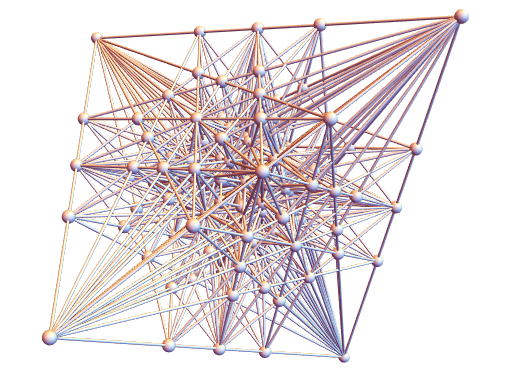✕ ```Module[{majorLines, tetrahedral, base, points, lines}, majorLines[pts_] := ((Drop[#1, -1] &) /@ #1 &) /@ Select[(Union[Flatten[#1, 1]] &) /@ SplitBy[SortBy[Subsets[(Append[#1, 1] &) /@ pts, {2}], RowReduce], RowReduce], Length[#1] > 4 &]; tetrahedral[{a_, b_, c_}] := Union[{{a, b, c}, {a, -b, -c}, {b, c, a}, {b, -c, -a}, {c, a, b}, {c, -a, -b}, {-c, a, -b}, {-c, -a, b}, {-b, c, -a}, {-b, -c, a}, {-a, b, -c}, {-a, -b, c}}]; base = {{0, 0, 0}, {180, 180, 180}, {252, 252, -252}, {420, 420, 420}, {1260, 1260, -1260}, {0, 0, 420}, {0, 0, 1260}, {0, 180, 360}, {0, 315, 315}, {0, 360, 180}, {0, 420, 840}, {0, 630, 630}, {0, 840, 420}, {140, 140, 420}, {180, 180, -540}, {252, 252, 756}, {420, 420, -1260}}; points = Union[Flatten[tetrahedral[#] & /@ base, 1]]; lines = majorLines[points]; Graphics3D[{Sphere[#, 50] & /@ points, Tube[Sort[#], 10] & /@ Select[lines, Length[#] == 5 &]}, Boxed -> False, ImageSize -> {500, 460}]]```

The 3D display is based on the following 2D solution, which has 25 points in 18 lines of 5 points. The numbers are barycentric coordinates. To use point 231, separate the digits (2,3,1), divide by the total (2/6,3/6,1/6) and simplify (1/3,1/2,1/6). If the outer triangle has area 1, the point 231 extended to the outer edges will make triangles of area (1/3,1/2,1/6).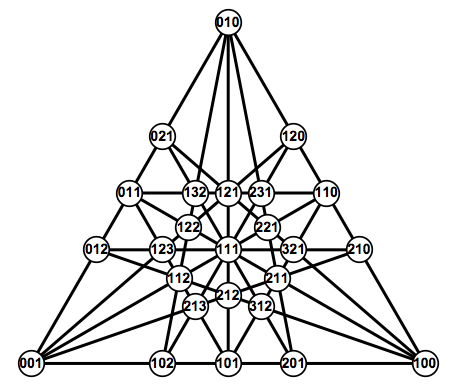✕ ```Module[{peggpoints, elkpoints, elklines, linecoords}, peggpoints = Sort[#/Total[#] & /@ Flatten[(Permutations /@ {{0, 0, 1}, {0, 1, 1}, {0, 1, 2}, {0, 4, 5}, {1, 1, 2}, {1, 2, 2}, {1, 2, 3}, {1, 2, 6}, {1, 4, 4}, {2, 2, 3}, {2, 2, 5}, {2, 3, 4}, {2, 3, 5}, {2, 5, 5}, {2, 6, 7}, {4, 5, 6}}), 1]]; elkpoints = Sort[#/Total[#] & /@ Flatten[(Permutations /@ {{1, 1, 1}, {0, 0, 1}, {1, 2, 3}, {1, 1, 2}, {0, 1, 1}, {1, 2, 2}, {0, 1, 2}}), 1]]; elklines = First /@ Select[ SortBy[Tally[BaryLiner[#] & /@ Subsets[elkpoints, {2}]], Last], Last[#] > 4 &]; linecoords = Table[FromBarycentrics[{#[], #[]}, tri] & /@ Select[elkpoints, elklines[[n]].# == 0 &], {n, 1, 18}]; Graphics[{AbsoluteThickness, Line /@ linecoords, With[{coord = FromBarycentrics[{#[], #[]}, tri]}, {Black, Disk[coord, .12], White, Disk[coord, .105], Black, Style[Text[StringJoin[ToString /@ (# (Max[Denominator[#]]))], coord], 14, Bold]}] & /@ elkpoints}, ImageSize -> {520, 450}]]```

A further exploration of this is at Extreme Orchards for Gardner. There, I ask if a self-dual configuration exists where the point set is identical to the line set. I managed to find the following 24-point 3-configuration. The numbers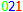represent {0,2,–1}, with blue = positive, red = negative and green = zero. In barycentric coordinates, a line {a,b,c} is on point {d,e,f} if the dot product {a,b,c}.{d,e,f}==0. For point {0,2,–1}, the lines {{–1,1,2},{–1,2,4},{0,1,2}} go through that point. Similarly, for line {0,2,–1}, the points {{–1,1,2},{–1,2,4},{0,1,2}} are on that line. The set of 24 points is identical to the set of 24 lines.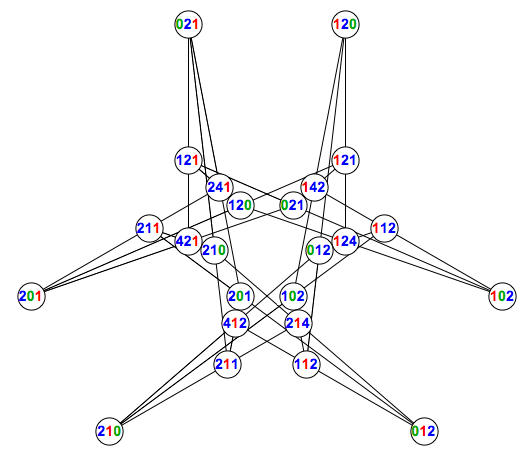✕ ```FromBarycentrics[{m_, n_, o_}, {{x1_, y1_}, {x2_, y2_}, {x3_, y3_}}] := {m*x1 + n*x2 + (1 - m - n)*x3, m*y1 + n*y2 + (1 - m - n)*y3}; tri = Reverse[{{Sqrt/2, -(1/2)}, {0, 1}, {-(Sqrt/2), -(1/2)}}]; With[{full = Union[Flatten[{#, RotateRight[#, 1], RotateLeft[#, 1]} & /@ {{-1, 0, 2}, {-1, 1, 2}, {-1, 2, 0}, {-1, 2, 1}, {-1, 2, 4}, {-1, 4, 2}, {0, 1, 2}, {0, 2, 1}}, 1]]}, Graphics[{EdgeForm[Black], Tooltip[Line[#[]], Style[Row[ Switch[Sign[#], -1, Style[ToString[Abs[#]], Red], 0, Style[ToString[Abs[#]], Darker[Green]], 1, Style[ToString[Abs[#]], Blue]] & /@ #[]], 16, Bold]] & /@ Table[{full[[k]], Sort[FromBarycentrics[#/Total[#], tri] & /@ Select[full, full[[k]].# == 0 &]]}, {k, 1, Length[full]}], White, {Disk[FromBarycentrics[#/Total[#], tri], .15], Black, Style[Text[ Row[Switch[Sign[#], -1, Style[ToString[Abs[#]], Red], 0, Style[ToString[Abs[#]], Darker[Green]], 1, Style[ToString[Abs[#]], Blue]] & /@ #], FromBarycentrics[#/Total[#], tri]], 14, Bold]} & /@ full}, ImageSize -> 520]]```

With a longer computer run, I found an order-27, self-dual 4-configuration where the points and lines have the same set of barycentric coordinates.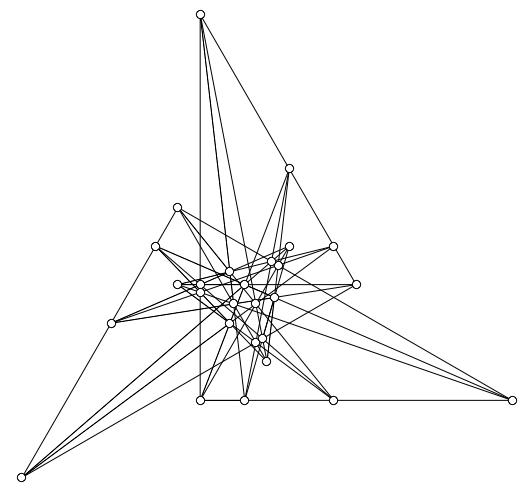✕ ```With[{full = Union[Flatten[{#, RotateRight[#, 1], RotateLeft[#, 1]} & /@ {{-2, -1, 4}, {-2, 1, 3}, {-1, 1, 1}, {-1, 2, 0}, {-1, 2, 1}, {-1, 3, 2}, {-1, 4, 2}, {0, 1, 2}, {1, 1, 2}}, 1]]}, Graphics[{EdgeForm[Black], Tooltip[Line[#[]], Style[Row[ Switch[Sign[#], -1, Style[ToString[Abs[#]], Red], 0, Style[ToString[Abs[#]], Darker[Green]], 1, Style[ToString[Abs[#]], Blue]] & /@ #[]], 16, Bold]] & /@ Table[{full[[k]], Sort[FromBarycentrics[#/Total[#], tri] & /@ Select[full, full[[k]].# == 0 &]]}, {k, 1, Length[full]}], White, {Tooltip[Disk[FromBarycentrics[#/Total[#], tri], .08], Style[Row[ Switch[Sign[#], -1, Style[ToString[Abs[#]], Red], 0, Style[ToString[Abs[#]], Darker[Green]], 1, Style[ToString[Abs[#]], Blue]] & /@ #], 16, Bold]]} & /@ full}, ImageSize -> 520]]```

And now back to the mathematics of three-in-a-row, frequently known as elliptic curve theory, but I’ll mostly be veering into geometry.

## Cubic Curves and Zero-Sum Geometries

In the cubic curve given by y = x3, all the triples from {–7,–6,…,7} that sum to zero happen to be on a straight line. The Table values are adjusted so that the aspect ratio will be reasonable.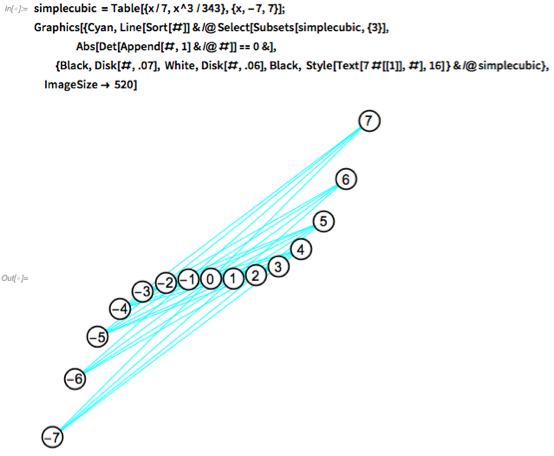✕ ```simplecubic = Table[{x/7, x^3 /343}, {x, -7, 7}]; Graphics[{Cyan, Line[Sort[#]] & /@ Select[Subsets[simplecubic, {3}], Abs[Det[Append[#, 1] & /@ #]] == 0 &], {Black, Disk[#, .07], White, Disk[#, .06], Black, Style[Text[7 #[], #], 16] } & /@ simplecubic}, ImageSize -> 520]```

For example, (2,3,–5) has a zero-sum. For the cubic curve, those numbers are at coordinates (2,8), (3,27) and (–5,–125), which are on a line. The triple (–∛2, –∛3, ∛2 + ∛3) also sums to zero and the corresponding points also lie on a straight line, but ignore that: restrict the coordinates to integers. With the curve y = x3, all of the integers can be plotted. Any triple of integers that sums to zero is on a straight line.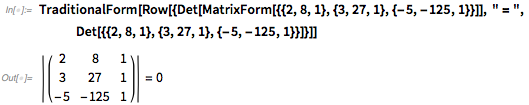✕ ```TraditionalForm[ Row[{Det[MatrixForm[{{2, 8, 1}, {3, 27, 1}, {-5, -125, 1}}]], " = ", Det[{{2, 8, 1}, {3, 27, 1}, {-5, -125, 1}}]}]]```

We can use the concept behind the cubic curve to make a rotationally symmetric zero-sum geometry around 0. Let blue, red and green represent positive, negative and zero values. Start with: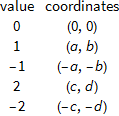To place the values 3 and 4, variables e and f are needed. The positions of all subsequent points up to infinity are forced.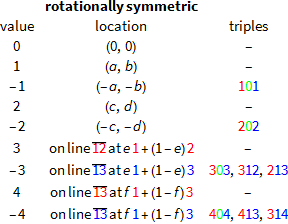Note that e and f should not be 0 or 1, since that would cause all subsequent points to overlap on the first five points.

Instead of building around 0, values can instead be reflected in the y = x diagonal to make a mirror-symmetric zero-sum geometry.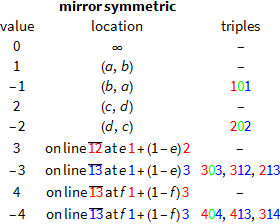Skew symmetry is also possible with the addition of variables (m,n).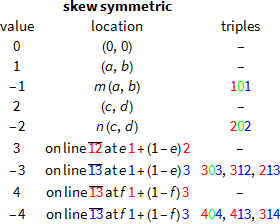The six variables (a,b,c,d,e,f) completely determine as many points as you like with rotational symmetry about (0,0) or mirror symmetry about the line y = x. Adding the variables (m,n) allows for a skew symmetry where the lines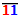and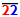intersect at (0,0). In the Manipulate, move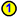to change (a,b) and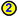to change (c,d). Move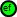horizontally to change e and vertically to change f. For skew symmetry, move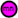to change the placements of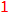and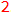.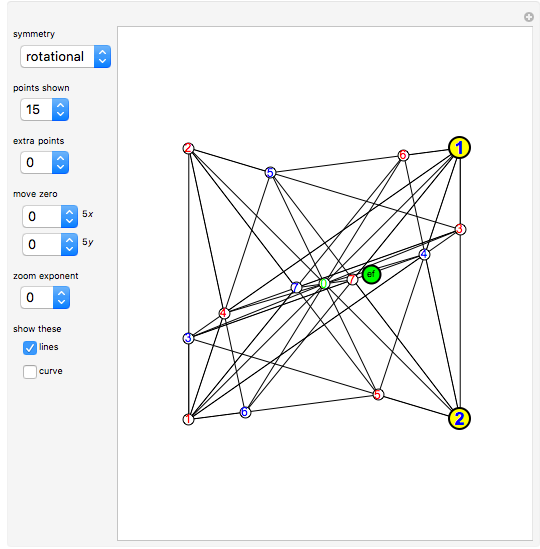✕ ```Manipulate[ Module[{ halfpoints, triples, initialpoints, pts2, candidate2}, halfpoints = Ceiling[(numberofpoints - 1)/2]; triples = Select[Subsets[Range[-halfpoints, halfpoints], {3}], Total[#] == 0 &]; initialpoints = rotational /. Thread[{a, b, c, d, e, f} -> Flatten[{ab, cd, ef}]]; If[symmetry == "mirror", initialpoints = mirror /. Thread[{a, b, c, d, e, f} -> Flatten[{ab, cd, ef}]]]; If[symmetry == "skew", initialpoints = skew /. Thread[{a, b, c, d, e, f, m, n} -> Flatten[{ab, cd, ef, mn}]]]; pts2 = Join[initialpoints, Table[{{0, 0}, {0, 0}}, {46}]]; Do[pts2[[ index]] = (LineIntersectionPoint33[{{pts2[[1, #]], pts2[[index - 1, #]]}, {pts2[[2, #]], pts2[[index - 2, #]]}}] & /@ {2, 1}), {index, 5, 50}]; If[showcurve, candidate2 = NinePointCubic2[First /@ Take[pts2, 9]], Sequence @@ {}]; Graphics[{ EdgeForm[Black], If[showcurve, ContourPlot[Evaluate[{candidate2 == 0}], {x, -3, 3}, {y, -3, 3}, PlotPoints -> 15][], Sequence @@ {}], If[showlines, If[symmetry == "mirror", {Black, Line[pts2[[Abs[#], (3 - Sign[#])/2 ]] & /@ #] & /@ Select[triples, Not[MemberQ[#, 0]] &], Green, InfiniteLine[ pts2[[Abs[#], (3 - Sign[#])/ 2 ]] & /@ #] & /@ (Drop[#, {2}] & /@ Select[triples, MemberQ[#, 0] &])}, {Black, Line[If[# == 0, {0, 0}, pts2[[Abs[#], (3 - Sign[#])/2 ]]] & /@ #] & /@ triples}], Sequence @@ {}], If[extrapoints > 0, Table[{White, Disk[pts2[[n, index]], .03]}, {n, halfpoints + 1, halfpoints + extrapoints}, {index, 1, 2}], Sequence @@ {}], Table[{White, Disk[pts2[[n, index]], .08], {Blue, Red}[[index]], Style[Text[n, pts2[[n, index]]] , 12]}, {n, halfpoints, 1, -1}, {index, 1, 2}], If[symmetry != "mirror", {White, Disk[{0, 0}, .08], Green, Style[Text[0, {0, 0}] , 12]}, Sequence @@ {}], Inset[\!\(\* GraphicsBox[ {RGBColor[1, 1, 0], EdgeForm[{GrayLevel, Thickness[Large]}], DiskBox[{0, 0}], {RGBColor[0, 0, 1], StyleBox[InsetBox["\<\"1\"\>", {0.05, -0.05}], StripOnInput->False, FontSize->18, FontWeight->Bold]}}, ImageSize->{24, 24}]\), ab], Inset[\!\(\* GraphicsBox[ {RGBColor[1, 1, 0], EdgeForm[{GrayLevel, Thickness[Large]}], DiskBox[{0, 0}], {RGBColor[0, 0, 1], StyleBox[InsetBox["\<\"2\"\>", {0.07, -0.05}], StripOnInput->False, FontSize->18, FontWeight->Bold]}}, ImageSize->{24, 24}]\), cd], Inset[\!\(\* GraphicsBox[ {RGBColor[0, 1, 0], EdgeForm[{GrayLevel, Thickness[Large]}], DiskBox[{0, 0}], {GrayLevel, StyleBox[InsetBox["\<\"ef\"\>", {0, 0}], StripOnInput->False, FontSize->9]}}, ImageSize->{21, 21}]\), ef], If[symmetry == "skew", Inset[\!\(\* GraphicsBox[ {RGBColor[1, 0, 1], EdgeForm[{GrayLevel, Thickness[Large]}], DiskBox[{0, 0}], {GrayLevel, StyleBox[InsetBox["\<\"mn\"\>", {0, 0}], StripOnInput->False, FontSize->9]}}, ImageSize->{21, 21}]\), mn], Sequence @@ {}]}, ImageSize -> {380, 480}, PlotRange -> Dynamic[(3/2)^zoom {{-2.8, 2.8} - zx/5, {-2.5, 2.5} - zy/5}]]], {{ab, {2, 2}}, {-2.4, -2.4}, {2.4, 2.4}, ControlType -> Locator, Appearance -> None}, {{cd, {2, -2}}, {-2.4, -2.4}, {2.4, 2.4}, ControlType -> Locator, Appearance -> None}, {{ef, {.7, .13}}, {-2.4, -2.4}, {2.4, 2.4}, ControlType -> Locator, Appearance -> None}, {{mn, {-2.00, -0.5}}, {-2.4, -2.4}, {2.4, 2.4}, ControlType -> Locator, Appearance -> None}, "symmetry", Row[{Control@{{symmetry, "rotational", ""}, {"rotational", "mirror", "skew"}, ControlType -> PopupMenu}}], "", "points shown", {{numberofpoints, 15, ""}, 5, 30, 2, ControlType -> PopupMenu}, "", "extra points", {{extrapoints, 0, ""}, 0, 20, 1, ControlType -> PopupMenu}, "", "move zero", Row[{Control@{{zx, 0, ""}, -10, 10, 1, ControlType -> PopupMenu}, " 5", Style["x", Italic]}], Row[{Control@{{zy, 0, ""}, -10, 10, 1, ControlType -> PopupMenu}, " 5", Style["y", Italic]}], "", "zoom exponent", {{zoom, 0, ""}, -2, 3, 1, ControlType -> PopupMenu}, "", "show these", Row[{Control@{{showlines, True, ""}, {True, False}}, "lines"}], Row[{Control@{{showcurve, False, ""}, {True, False}}, "curve"}], TrackedSymbols :> {ab, cd, ef, mn, zx, zy, symmetry, numberofpoints, extrapoints, zoom}, ControlPlacement -> Left, Initialization :> ( Clear[a]; Clear[b]; Clear[c]; Clear[d]; Clear[e]; Clear[f]; Clear[m]; Clear[n]; NinePointCubic2[pts3_] := Module[{makeRow2, cubic2, poly2, coeff2, nonzero, candidate}, If[Min[ Total[Abs[RowReduce[#][]]] & /@ Subsets[Append[#, 1] & /@ pts3, {4}]] > 0, makeRow2[{x_, y_}] := {1, x, x^2, x^3, y, y x, y x^2, y^2, y^2 x, y^3}; cubic2[x_, y_][p_] := Det[makeRow2 /@ Join[{{x, y}}, p]]; poly2 = cubic2[x, y][pts3]; coeff2 = Flatten[CoefficientList[poly2, {y, x}]]; nonzero = First[Select[coeff2, Abs[#] > 0 &]]; candidate = Expand[Simplify[ poly2/nonzero]]; If[Length[FactorList[candidate]] > 2, "degenerate", candidate], "degenerate"]]; LineIntersectionPoint33[{{a_, b_}, {c_, d_}}] := ( Det[{a, b}] (c - d) - Det[{c, d}] (a - b))/Det[{a - b, c - d}]; skew = {{{a, b}, {a m, b m}}, {{c, d}, {c n, d n}}, {{a e m - c (-1 + e) n, b e m - d (-1 + e) n}, {( a e m + c n - c e n)/(e m + n - e n), (b e m + d n - d e n)/( e m + n - e n)}}, {{a f m - ((-1 + f) (a e m - c (-1 + e) n))/( e (m - n) + n), b f m - ((-1 + f) (b e m - d (-1 + e) n))/(e (m - n) + n)}, {( c (-1 + e) (-1 + f) n + a m (e + e f (-1 + m - n) + f n))/( 1 + f (-1 + e m (m - n) + m n)), ( d (-1 + e) (-1 + f) n + b m (e + e f (-1 + m - n) + f n))/( 1 + f (-1 + e m (m - n) + m n))}}}; rotational = {#, -#} & /@ {{a, b}, {c, d}, {c (-1 + e) - a e, d (-1 + e) - b e}, {c (-1 + e) (-1 + f) + a (e - (1 + e) f), d (-1 + e) (-1 + f) + b (e - (1 + e) f)}}; mirror = {#, Reverse[#]} & /@ {{a, b}, {c, d}, {d (1 - e) + b e, c (1 - e) + a e}, {(c (1 - e) + a e) (1 - f) + b f, (d (1 - e) + b e) (1 - f) + a f}};), SynchronousInitialization -> False, SaveDefinitions -> True]```

In the rotationally symmetric construction, point 7 can be derived by finding the intersection of lines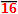,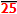and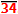.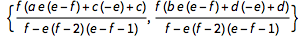✕ ```TraditionalForm[ FullSimplify[{h zerosumgeometrysymmetric[[2, 2]] + (1 - h) zerosumgeometrysymmetric[[5, 2]] } /. Solve[h zerosumgeometrysymmetric[[2, 2]] + (1 - h) zerosumgeometrysymmetric[[5, 2]] == j zerosumgeometrysymmetric[[3, 2]] + (1 - j) zerosumgeometrysymmetric[[4, 2]] , {h, j}][[ 1]]][]]```

The simple cubic had 15 points 7 to 7 producing 25 lines. That falls short of the record 31 lines. Is there a way to get 6 more lines? Notice 6 triples with a sum of 0 modulus 15: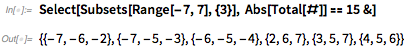✕ `Select[Subsets[Range[-7, 7], {3}], Abs[Total[#]] == 15 &]`

We can build up the triangle area matrices for those sets of points. If the determinant is zero, the points are on a straight line.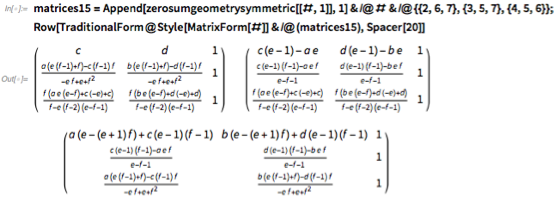✕ ```matrices15 = Append[zerosumgeometrysymmetric[[#, 1]], 1] & /@ # & /@ {{2, 6, 7}, {3, 5, 7}, {4, 5, 6}}; Row[TraditionalForm@Style[MatrixForm[#]] & /@ (matrices15), Spacer]```

Factor each determinant and hope to find a shared factor other than bcad, which puts all points on the same line. It turns out the determinants have —e + e2 + fe f + f2e f2 + f3 as a shared factor.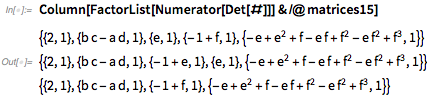✕ `Column[FactorList[Numerator[Det[#]]] & /@ matrices15]`

Are there any nice solutions for —e + e2 + fe f + f2e f2 + f3 = 0? Turns out letting e=Φ (the golden ratio) allows f = –1.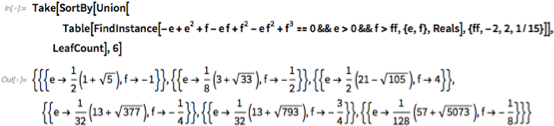✕ ```Take[SortBy[Union[ Table[FindInstance[-e + e^2 + f - e f + f^2 - e f^2 + f^3 == 0 && e > 0 && f > ff, {e, f}, Reals], {ff, -2, 2, 1/15}]], LeafCount], 6]```

Here’s what happens with base points (a,b) = (1,1), (c,d) = (1,–1) and that value of (e,f).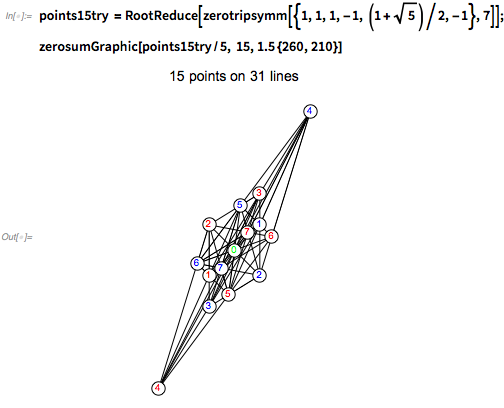✕ ```points15try = RootReduce[zerotripsymm[{1, 1, 1, -1, (1 + Sqrt)/2, -1}, 7]]; zerosumGraphic[points15try/5, 15, 1.5 {260, 210}]```

The solution’s convex hull is determined by points 4 and 2, so those points can be moved to make the solution more elegant.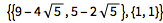✕ ```RootReduce[({{w, x}, {y, z}} /. Solve[{{{w, x}, {y, z}}.points15try[[2, 1]] == {1, 1}, {{w, x}, {y, z}}.points15try[[4, 1]] == {-1, 1}}][[ 1]]).# & /@ {points15try[[1, 1]], points15try[[2, 1]]}]```

The values for (a,b,c,d) do not need to be exact, so we can find the nearest rational values.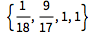✕ ```nearestRational[#, 20] & /@ Flatten[{{9 - 4 Sqrt, 5 - 2 Sqrt}, {1, 1}}]```

That leads to an elegant-looking solution for the 15-tree problem. There are 31 lines of 3 points, each a triple that sums to 0 (mod 15).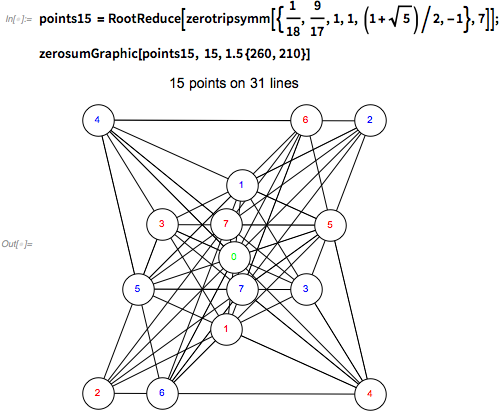✕ ```points15 = RootReduce[zerotripsymm[{1/18, 9/17, 1, 1, (1 + Sqrt)/2, -1}, 7]]; zerosumGraphic[points15, 15, 1.5 {260, 210}]```

The 14-point version leads to polynomial equation 2e – 2e2f + e f + ee f2 = 0, which has the nice solution {e->1/2,f-> (–1+√17)/4}. A point at infinity is needed for an even number of points with this method.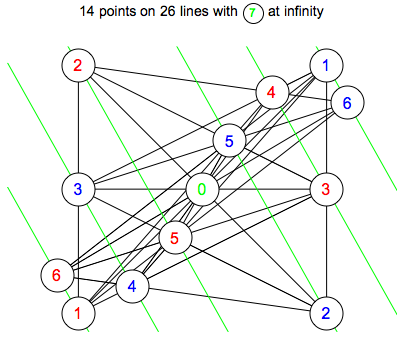✕ ```{{{1, 1}, {-1, -1}}, {{1, -1}, {-1, 1}}, {{-1, 0}, {1, 0}}, {{1/2 (3 - Sqrt), 1/4 (1 - Sqrt)}, {1/2 (-3 + Sqrt), 1/4 (-1 + Sqrt)}}, {{1/4 (5 - Sqrt), 1/8 (-1 + Sqrt)}, {1/4 (-5 + Sqrt), 1/8 (1 - Sqrt)}}, {{1/8 (-3 + 3 Sqrt), 1/16 (7 + Sqrt)}, {1/8 (3 - 3 Sqrt), 1/16 (-7 - Sqrt)}}}```

The solution on 15 points can be tweaked to give a match for the 16-point, 37-line solution in various ways. The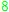is not particularly meaningful here. The last example is done with skew symmetry, even though it seems the same.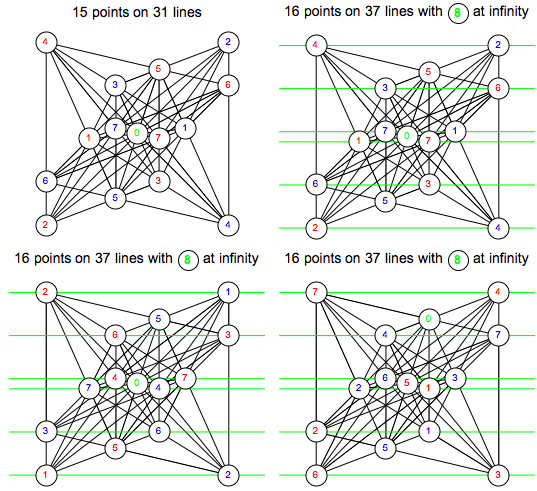✕ ```Grid[Partition[{zerosumGraphic[ zerotripsymm[{5 - 2 Sqrt, 9 - 4 Sqrt, 1, 1, 1/2 (1 + Sqrt), -1}, 7], 15, {260, 210}], zerosumGraphic[ zerotripsymm[{5 - 2 Sqrt, 9 - 4 Sqrt, 1, 1, 1/2 (1 + Sqrt), -1}, 7], 16, {260, 210}], zerosumGraphic[ zerotripsymm[{1, 1, 1, -1, 3 - Sqrt, 1/2 (3 - Sqrt)}, 7], 16, {260, 210}], zerosumGraphic[ RootReduce[ zerotripskew[{0, 1 - Sqrt, -3 + Sqrt, -3 + Sqrt, -1 + Sqrt, 1/2 (1 + Sqrt), 1/2 (-1 + Sqrt), 1/2 (1 + Sqrt)}, 7]], 16, {260, 210}]}, 2]]```

The first solution is a special case of the 15-solution with an abnormal amount of parallelism, enough to match the sporadic 16-point solution. How did I find it?

## Orchard-Planting Polynomials

Here are coordinates for the positive points up to 4 in the mirror-symmetric and skew-symmetric cases. They quickly get more complicated.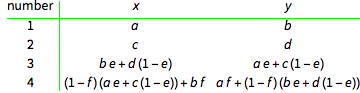✕ ```TraditionalForm@ Grid[Prepend[ Transpose[ Prepend[Transpose[First /@ Take[zerosumgeometrymirror, 4]], Range[1, 4]]], {"number", x, y}], Dividers -> {{2 -> Green}, {2 -> Green}}]```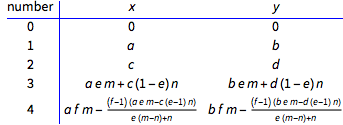✕ ```TraditionalForm@ Grid[Prepend[ Transpose[ Prepend[Transpose[ Prepend[First /@ Take[zerosumgeometryskew, 4], {0, 0}]], Range[0, 4]]], {"number", x, y}], Dividers -> {{2 -> Blue}, {2 -> Blue}}]```

Here are coordinates for the positive points up to 7 in the rotationally symmetric case. These are more tractable, so I focused on them.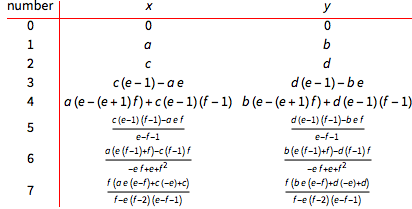✕ ```TraditionalForm@ Grid[Prepend[ Transpose[ Prepend[Transpose[ Prepend[First /@ Take[zerosumgeometrysymmetric, 7], {0, 0}]], Range[0, 7]]], {"number", x, y}], Dividers -> {{2 -> Red}, {2 -> Red}}]```

For 14 and 15 points, the polynomials 2e – 2e2f + e f + e2 fe f2 and —e + e2 + fe f + f2e f2 + f3 appeared almost magically to solve the problem. Why did that happen? I have no idea, but it always seems to work. I’ll call these orchard-planting polynomials. It’s possible that they’ve never been used before to produce elegant solutions for this problem, because we would have seen them. Here are the next few orchard-planting polynomials. As a reminder, these are shared factors of the determinants generated by forcing triples modulo p to be lines.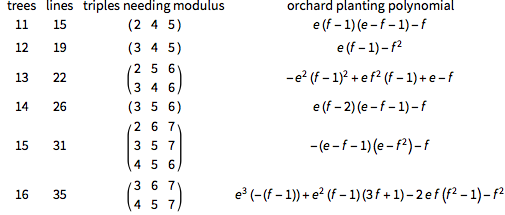✕ ```Monitor[TraditionalForm@Grid[Prepend[Table[ With[{subs = Select[Subsets[Range[-Floor[n/2], Floor[n/2]], {3}], Mod[ Abs[Total[#]], n ] == 0 && Not[MemberQ[#, -(n/2)]] &]}, {n, Length[subs], Select[subs, Min[#] > 0 && Max[#] < 13 && Max[#] < n/2 &], Last[SortBy[ Apply[Intersection, (First[Sort[FullSimplify[{#, -#}]]] & /@ First /@ FactorList[Numerator[#]] & /@ Expand[Det[ Append[zerosumgeometrysymmetric[[#, 1]], 1] & /@ #] & /@ Select[subs, Min[#] > 0 && Max[#] < 13 && Max[#] < n/2 &]])], LeafCount]]}], {n, 11, 16}], {"trees", "lines", "triples needing modulus", "orchard planting polynomial"}]], n]```

Here is the major step for the solution of 14 trees. The item showing up in the numerator generated by (3,5,6) happens to be the denominator of item 7 = (3 + 5 + 6)/2.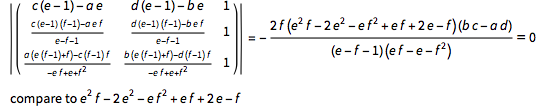✕ ```With[{mat = Append[zerosumgeometrysymmetric[[#, 1]], 1] & /@ {3, 5, 6}}, TraditionalForm[ Row[{Det[MatrixForm[mat]], " = ", Factor[Det[mat]] == 0, "\n compare to ", Expand[-Denominator[zerosumgeometrysymmetric[[7, 1, 1]] ]]}]]]```

But I should have expected this. The solution for 18 points is next. The point 9 is at infinity! Therefore, level 9 needs 1/0 to work properly.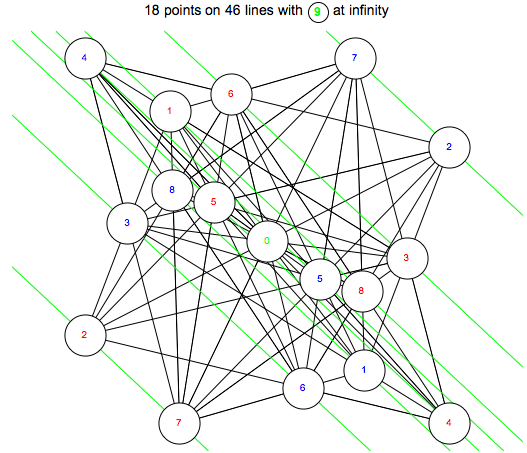✕ ```zerosumGraphic[zerotripsymm[orchardsolutions[[18, 4]], 8], 18, 2 {260, 210}]```

Here's a contour plot of all the orchard-planting polynomials up to order 28. The number values give the location of a particularly elegant solution for that number of points.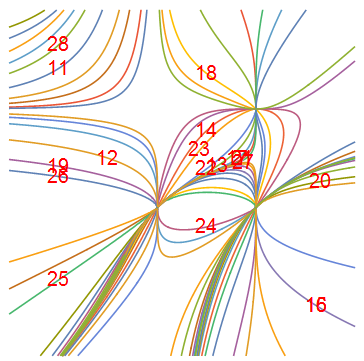✕ ```allorchardpolynomials = Table[orchardsolutions[[ff, 5]] == 0, {ff, 11, 27, 2}]; Graphics[{ContourPlot[ Evaluate[allorchardpolynomials], {e, -3/2, 2}, {f, -3/2, 2}, PlotPoints -> 100][], Red, Table[Style[Text[n, Take[orchardsolutions[[n, 4]], -2]], 20], {n, 11, 28}]}]```

Recall from the construction that e and f should not be 0 or 1, since that would cause all subsequent points to overlap on the first five points, causing degeneracy. The curves intersect at these values.

We can also plot the locations where the e f values lead to lines of two points having the same slope. Forcing parallelism leads to hundreds of extra curves. Do you see the lower-right corner where the green curve is passing through many black curves? That's the location of the sporadic 16-point solution. It's right there!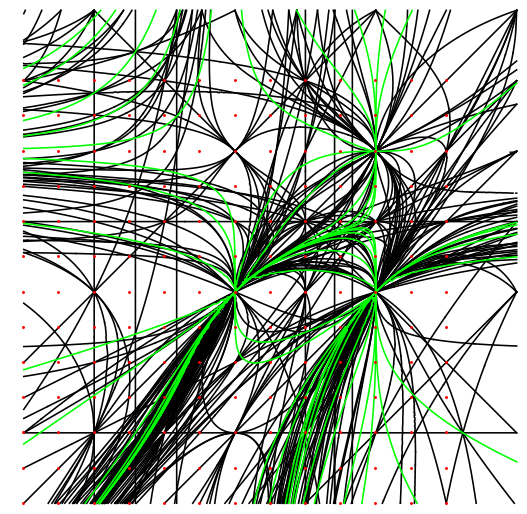✕ ```slope[{{x1_, y1_}, {x2_, y2_}}] := (y2 - y1)/(x2 - x1); theslopes = {# - 1, FullSimplify[ slope[Prepend[ First /@ Take[zerosumgeometrysymmetric, 11], {0, 0}][[#]]]]} & /@ Subsets[Range[ 10], {2}]; sameslope = {#[[2, 1]], #[]} & /@ (Transpose /@ SplitBy[SortBy[{#[], #[[2, 1]] == Simplify[#[[2, 2]]]} & /@ ({#[], Flatten[#[]]} & /@ SortBy[ Flatten[Transpose[{Table[#[[ 1]], {Length[#[]]}], (List @@@ # & /@ #[[ 2]])}] & /@ Select[{#[], Solve[{#[[2, 1]] == #[[2, 2]], d != (b c)/a , e != 0, e != 1, f != 0, f != 1}]} & /@ Take[SortBy[(Transpose /@ Select[Subsets[theslopes, {2}], Length[Union[Flatten[First /@ #]]] == 4 &]), Total[Flatten[#[]]] &], 150], Length[StringPosition[ToString[FullForm[#[]]], "Complex"]] == 0 && Length[#[]] > 0 &], 1], Last]), Last], Last]); Graphics[{Table[ ContourPlot[ Evaluate[sameslope[[n, 1]]], {e, -3/2, 2}, {f, -3/2, 2}, PlotPoints -> 50, ContourStyle -> Black][], {n, 1, 162}], Red, Table[ContourPlot[ Evaluate[allorchardpolynomials[[n]]], {e, -3/2, 2}, {f, -3/2, 2}, PlotPoints -> 50, ContourStyle -> Green][], {n, 1, 18}], Tooltip[Point[#], #] & /@ Tuples[Range[-6, 6]/4, {2}] }]```

That's my way to find sporadic solutions. The mirror and skew plots have added levels of messiness sufficient to defy my current ability to analyze them.

Is there an easy way to generate these polynomials? I have no idea. Here are plots of their coefficient arrays.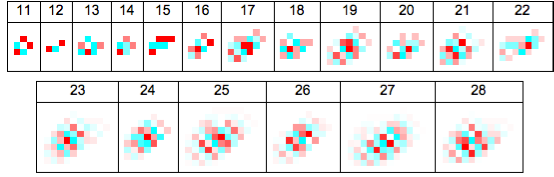✕ ```Column[{Text@ Grid[{Range[11, 22], With[{array = CoefficientList[#, {e, f}]}, With[{rule = Thread[Apply[Range, MinMax[Flatten[array]]] -> Join[Reverse[ Table[ RGBColor[1, 1 - z/Abs[Min[Flatten[array]]], 1 - z/Abs[Min[Flatten[array]]]], {z, 1, Abs[Min[Flatten[array]]]}]], {RGBColor[1, 1, 1]}, Table[ RGBColor[1 - z/Abs[Max[Flatten[array]]], 1, 1], {z, 1, Abs[Max[Flatten[array]]]}]]]}, ArrayPlot[array, ColorRules -> rule, ImageSize -> Reverse[Dimensions[array]] {7, 7}, Frame -> False ]]] & /@ (#[] & /@ Take[orchardsolutions, {11, 22}])}, Frame -> All], Text@Grid[{Range[23, 28], With[{array = CoefficientList[#, {e, f}]}, With[{rule = Thread[Apply[Range, MinMax[Flatten[array]]] -> Join[Reverse[ Table[ RGBColor[1, 1 - z/Abs[Min[Flatten[array]]], 1 - z/Abs[Min[Flatten[array]]]], {z, 1, Abs[Min[Flatten[array]]]}]], {RGBColor[1, 1, 1]}, Table[ RGBColor[1 - z/Abs[Max[Flatten[array]]], 1, 1], {z, 1, Abs[Max[Flatten[array]]]}]]]}, ArrayPlot[array, ColorRules -> rule, ImageSize -> Reverse[Dimensions[array]] {7, 7}, Frame -> False ]]] & /@ (#[] & /@ Take[orchardsolutions, {23, 28}])}, Frame -> All]}, Alignment -> Center]```

## Graphics of Orchard Solutions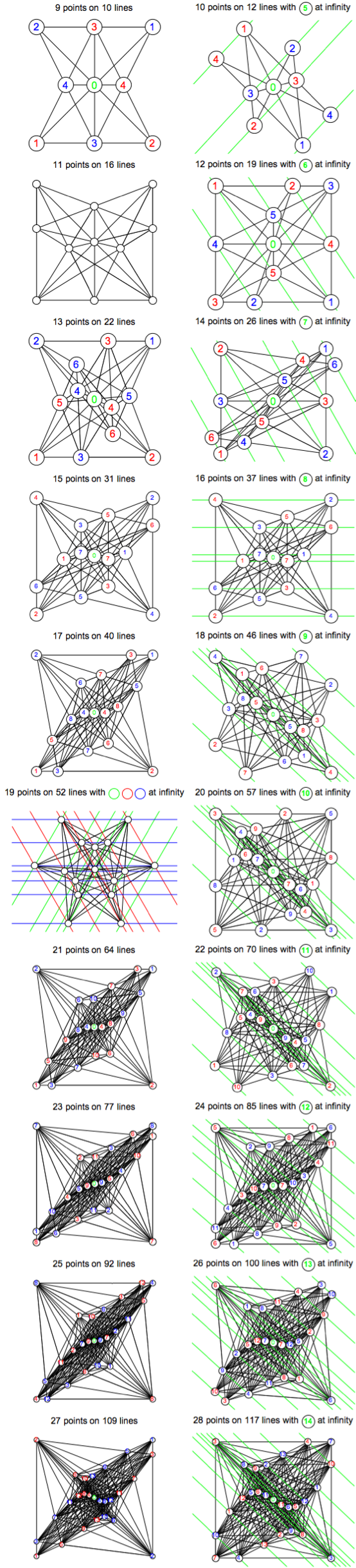✕ ```Grid[Partition[Table[Quiet@ zerosumGraphic[ If[orchardsolutions[[n, 2]] > orchardsolutions[[n, 3]], orchardsolutions[[n, 6]], Quiet@zerotripsymm[orchardsolutions[[n, 4]], Floor[(n - 1)/2]]], n, {260, 210}], {n, 9, 28}], 2]]```

Download the full notebook to see all the code used for finding elegant-looking solutions.

## Unsolved Problems

Looking for unsolved problems of the orchard-planting variety? Here are several I suggest:

1. Do more sporadic solutions exist for the three-orchard problem?
2. Can 11- and 19-point solutions be found with partial zero-sum geometry?
3. Do better solutions exist for four-orchard solutions on 17 or more points?
4. Do smaller 3- and 4-configurations exist where the sets of the barycentric coordinates for the points and lines are the same?
5. Does a 5-configuration exist where the sets of the barycentric coordinates for the points and lines are the same?
6. What are best solutions for the five-orchard problem?
7. Is there a good method for generating orchard-planting polynomials?

And if you'd like to explore more recreational mathematics, check out some of the many entries on the Wolfram Demonstrations Project.